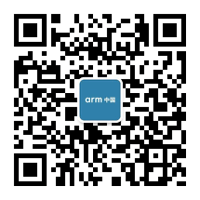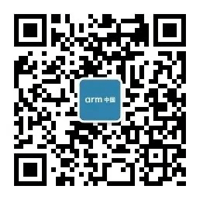# 用 HLS 实现 UART### 介绍

UART 是一种旧的串行通信机制，但仍在很多平台中使用。它在 HDL 语言中的实现并不棘手，可以被视为本科生的作业。在这里，我将通过这个例子来展示在 HLS 中实现它是多么容易和有趣。FPGA 板上的八个滑动开关用于输入数据字节（例如字母或符号的 ASCII 代码）。此外，UP 按钮用作发送控制键。``````bool delay(long long int n) {
static bool dummy = 0;
for (long long int j = 0; j < n; j++) {
#pragma HLS pipeline
dummy = !dummy;
}
return dummy;
}
void uart_baudrate_clock(bool &baudrate_clk) {
static bool s = 0;
s=!s;
baudrate_clk = s;
delay(5208);
}  ``````

``````void uart_data_transfer(bool &uart_tx, ap_uint<8> data, bool baud_rate_clock, bool start) {
static bool send_bit = 1;
static bool start_state = 0;
static bool transfer = 0;
static unsigned int count = 0;
static int state = 0;
ap_uint<10> d= ((bool)0b1, (ap_int<8>)data, (bool)0b0);
if (start == 1 && start_state == 0) {
transfer = 1;
start_state = 1;
count = 0;
}
if (start == 0 && start_state == 1) {
start_state = 0;
}
if (baud_rate_clock == 1 && state == 0 && transfer == 1) {
send_bit = d[count++];
if (count == 10) {
transfer = 0;
}
state = 1;
}
if (baud_rate_clock == 0 && state == 1) {
state = 0;
}
uart_tx = send_bit;
}
``````### 总结

4 阅读 290
##### 推荐阅读10387

458
FPGA Logic 二三事